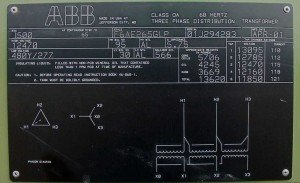Arc Flash & Electrical Power Training | Brainfiller

Arc Flash & Electrical Power Training by Jim Phillips | 800.874.8883 | +1 480.275.7451

# Short Circuit Calculations – Infinite Bus Method

Have you wondered is there a simpler way to calculate short circuit currents without a computer program? A very simplified method known by many as the “infinite bus” calculation method is a good way to approximate short circuit calculations.  This article takes you thought the steps with an example of the infinite bus calculation process.  If you would like to know how to perform more detailed short calculations which include the source impedance, conductor impedance and motor contribution in addition to the transformer impedance, Jim has a 4 DVD series that takes you through the entire short circuit calculation process complete with many examples and calculation worksheets.  Now, on with the article…

Three-phase transformers contain some valuable data on their nameplate such as the kVA rating, primary and secondary voltage, and percent impedance. With this minimal data, you can calculate the worst case short circuit current through a transformer. The calculation will provide the three-phase rms symmetrical short circuit current on the secondary bus of the transformer. This process only has two simple calculation steps:

Step 1 – Calculate the secondary full load current rating of the transformer:

• FLAsecondary = ( kVA3phase ) / kVL-L x Sqrt (3)

Step 2 – Calculate the short circuit current on the transformer secondary bus:

• SCAsecondary = x ( FLAsecondary x 100 ) / % ZHere is an example of the calculation:

The existing transformer is rated 1500 kVA with a secondary voltage of 480Y/277V and an impedance of 5.75%.

Step 1 – Calculate the secondary full load current rating of the transformer:

FLAsecondary = 1500 kVA / 0.48 kVL-L x Sqrt (3)

FLAsecondary = 1804 Amps

Step 2 – Calculate the short circuit current on the transformer secondary bus.

SCAsecondary = 1804 Amps x 100 / 5.75%

SCAsecondary = 31,374 Amps

All of the variables listed above are:

FLAsecondary = Secondary Full Load Amps

kVL-L = Secondary Voltage in kV
kVA3phase = Transformer Three Phase kVA
Sqrt (3) = Square Root of Three = (1.73)
% Z = Percent Impedance of Transformer
SCAsecondary = Short Circuit Amps at Secondary BusA few words of caution: The impedance of a transformer must be the actual nameplate and not an assumed value. Impedances of transformers that have not yet been built or tested can vary by + / – 7.5% of the specified impedance. The above calculation does not include something called motor contribution that is discuss in another article and also the calculation does not include any reduction in current due to the source or conductor impedance.

The infinite bus calculation method is generally NOT suitable for use with Arc Flash Studies since a lower short circuit current could result in the overcurrent protective device taking longer to operate resulting in a greater incident energy exposure.About Jim Phillips, P.E.: Electrical Power and Arc Flash Training Programs – For over 30 years, Jim Phillips has been helping tens of thousands of people around the world, understand electrical power system design, analysis, arc flash and electrical safety. Jim is Vice Chair of IEEE 1584 and International Chairman of IEC TC78 Live Working. He has developed a reputation for being one of the best trainers in the electric power industry, Learn More.